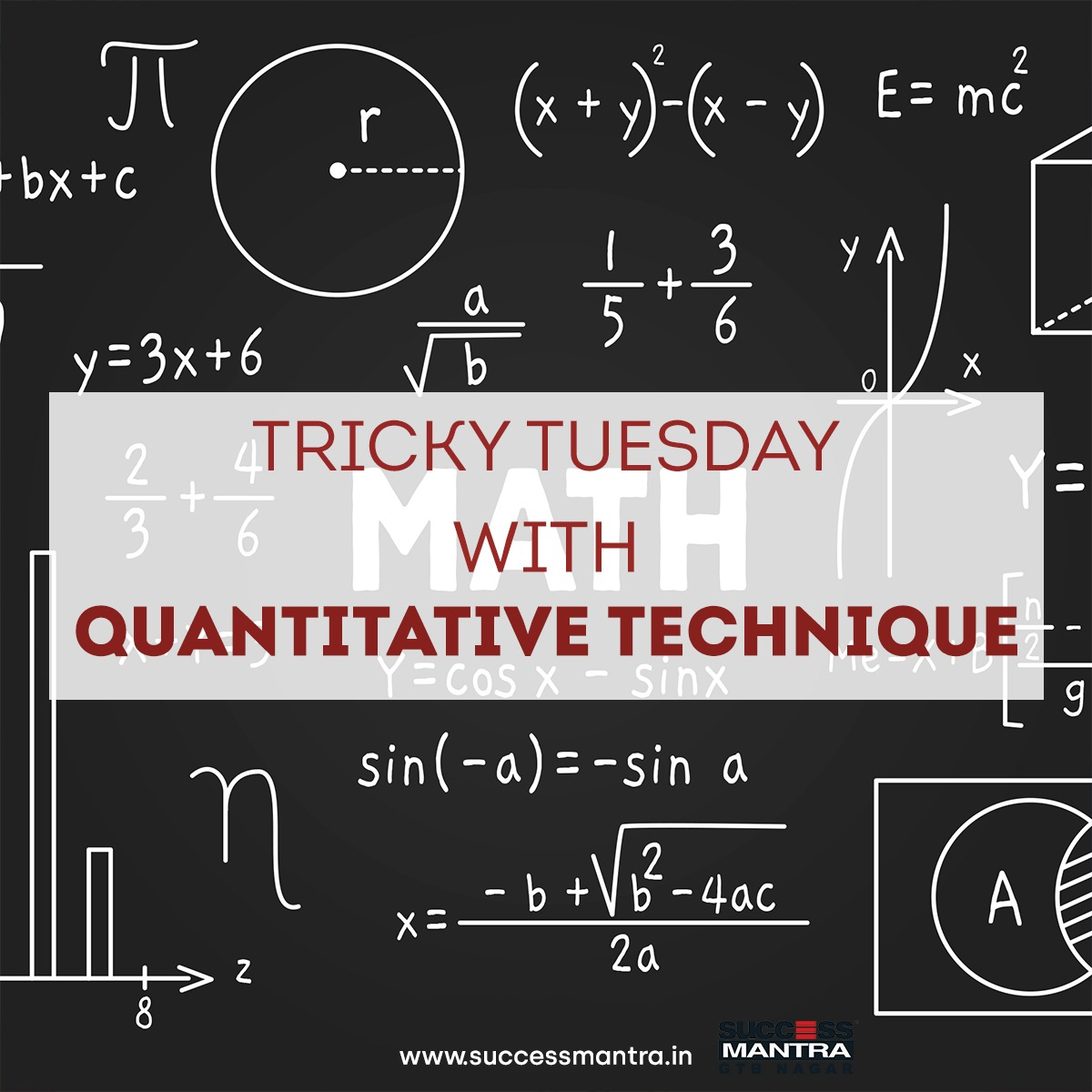## Questions On Quantitative Techniques SMQTQ0411. From a bank, Ram and Shyam together took a certain amount under simple interest and they lent the total amount to Mohan at 2% more simple interest. At the end of 4 years, the total money earned by Ram after paying the interest to the bank was Rs. 400 more than that of Shyam. From the bank, the total amount taken by Ram was how much more than that of Shyam?

A. Rs. 10000
B. Rs. 20000
C. Rs. 5000
D. Rs. 25000

2. Aman and Raghav are two friends. Aman started a business with an investment of Rs 7200, while Raghav puts 60% of his salary at 40% p.a simple interest for 6 months; Raghav takes the amount received after 6 months and joins Aman in the business. If Aman receives a profit of Rs. 2000 out of a total profit of Rs. 2900 at the end of 1 year, what was the original salary of Raghav?

A. Rs. 18000
B. Rs. 9400
C. Rs. 9000
D. Rs. 15000

3. Deepika deposited Rs. 1000 in a fund in 2019 which provides simple interest. The interest rate on the fund increases by 3% every year. If the interest rate at the time of amount deposit was 10%, find the interest earned by her after 9 years.

A. Rs. 1880
B. Rs. 1890
C. Rs. 2980
D. Rs. 1980

4. Rayan invested a total of Rs.49000 in two different schemes A and B. The scheme A which offers interest at a rate of 5% per annum and scheme B offers interest at a rate of 12%. If the total interest earned by Rayan after 1 year is Rs.4900 then find the sum invested in scheme B.

A. Rs. 34000
B. Rs. 19000
C. Rs. 35000
D. Rs. 14000

5. The salary of a man is Rs. 60000, from which he deposits x% amount at 13% simple interest. If the accumulated amount for the sum deposited after 3 years was Rs. 29190, then find the value of ‘x’.

A. 40
B. 35
C. 45
D. 50

6. Arnab deposited Rs. 14500 in SBI mutual fund which offers simple interest at the rate of 9%. The simple interest obtained from SBI is deposited in Birla Sun Life mutual fund at the rate of 12% simple interest. If the time period for depositing in SBI and Birla were 2 years and 5 years respectively, then find the total simple interest earned by Arnab.

A. Rs. 5248
B. Rs. 3856
C. Rs. 4176
D. Rs. 4462

1. C
2. C
3. D
4. C
5. B
6. C